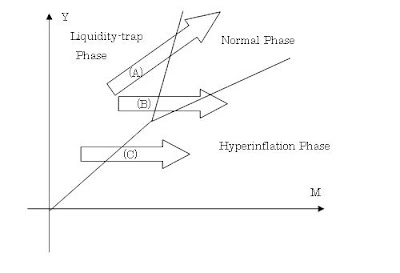# himaginary's blog

## 2011年6月12日日曜日

### Analogy between Gibbs free energy and excess demand for money

In thermal physics, Gibbs free energy is defined as follows:
G ≡ U - τσ + pV

where U is the internal energy, τ is the temperature, σ is the entropy, p is pressure, V is volume.

Therefore,
dG = dU - τdσ - σdτ + pdV + Vdp

Now: "Consider a system in thermal contact with a heat reservoir R1 at temperature τ and in mechanical contact with a pressure reservoir R2 that maintains the pressure p, but cannot exchange heat." (Kittel p.262)

In this system S, dτ=0 and dp=0, so
dGS ＝ dUS - τdσS + pdVS

In equilibrium, this equals zero for each set of (τ,p).

In monetary economics, excess demand for money can be derived from equation of exchange as follows:
G ≡ PY - MV

where P is the price, Y is the output, M is the money, V is the velocity of money.

Therefore,
dG = dPY + PdY - dMV - MdV

Now, consider a mediating system in financial contact with a central bank R1 that maintains the money M and in transactional contact with a real economy R2 that maintains the output Y.

In this system S, dM=0 and dY=0, so
dGS ＝ YdPS - MdVS

In equilibrium, this equals zero for each set of (M,Y).This analogy is the idea behind the previous post.

From this analogy, you can derive the monetary economics version of Clausius-Clapeyron relation. Note that in this analogy p(pressure) corresponds to Y(output), V(volume) corresponds to P(price), τ(temperature) corresponds to M(money), and σ(entropy) corresponds to V(velocity of money).

## 2011年6月11日土曜日

### Phase transition analogy of inflation

When zero interest rate is not enough to revive the depressed economy, quantitative easing is the next step for monetary policy to proceed. However, it is often the case that small increase in money supply is not enough. You'll need large increase in money supply to obtain some effect, but there is always risk of doing too much. That's what the ketchup theory of inflation warns us.

It may be interesting to think about this by analogy to latent heat in phase transition. Latent heat is what substance needs to complete phase transition (eg. from ice to water, liquid to gas). If the heat added is less than the latent heat, phase transition remains incomplete. So, in terms of quantitative easing, latent heat corresponds to the certain amount of money which is needed to change the state of economy.

Speaking of phase transition, it may be also interesting to draw phase diagram in monetary policy. If you compare liquidity-trap economy to solid phase, normal economy to liquid phase, and hyperinflation economy to gas phase, it goes like this:Here I took money supply M as X-axis(temperature in actual phase transition), and real output Y as Y-axis(pressure in actual phase transition).

Arrow (A) is what quantitative easing aims at. That is, by the appropriate increase in money, economy makes transition from liquidity-trap phase to normal phase.

However, if you do too much, the economy may jump over normal phase and move into hyperinflation phase(arrow (B)).
Or, real economy may not be strong enough to move back to normal state in the first place, and economy may move directly into hyperinflation phase(arrow (C)).
These scenarios are what inflationistas worry about.

BTW, I've also done some maths on this analogy, and will explain them in later post(s).

## 自己紹介

This blog is some thoughts on economics by a Japanese non-economist. Translated from my Japanese blog.